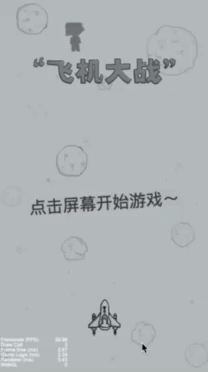# 微信飞机大战小游戏编写分享（上）## 2. 创建游戏项目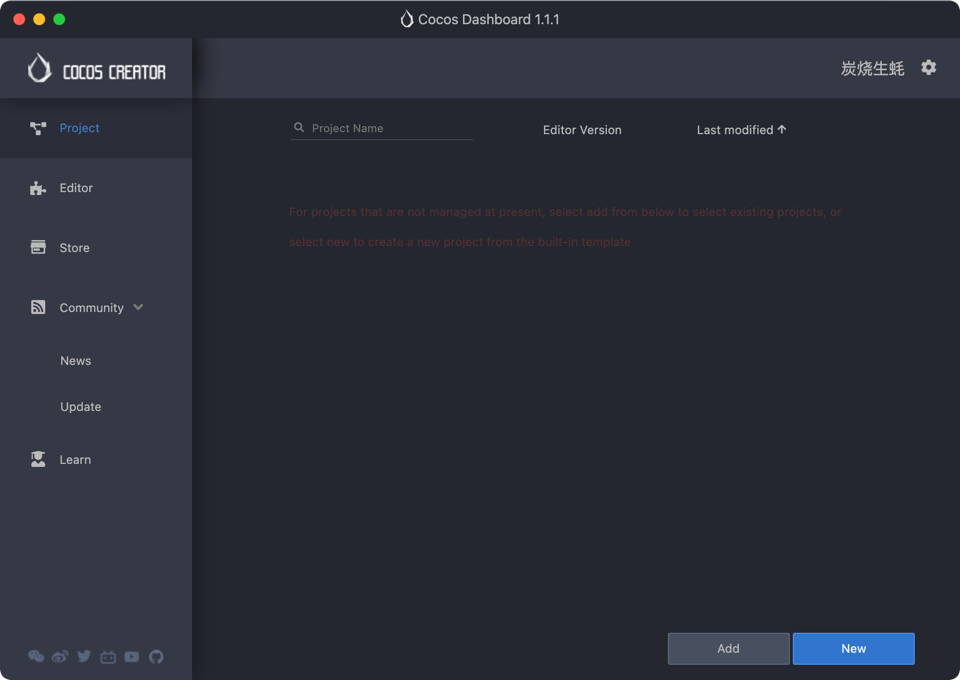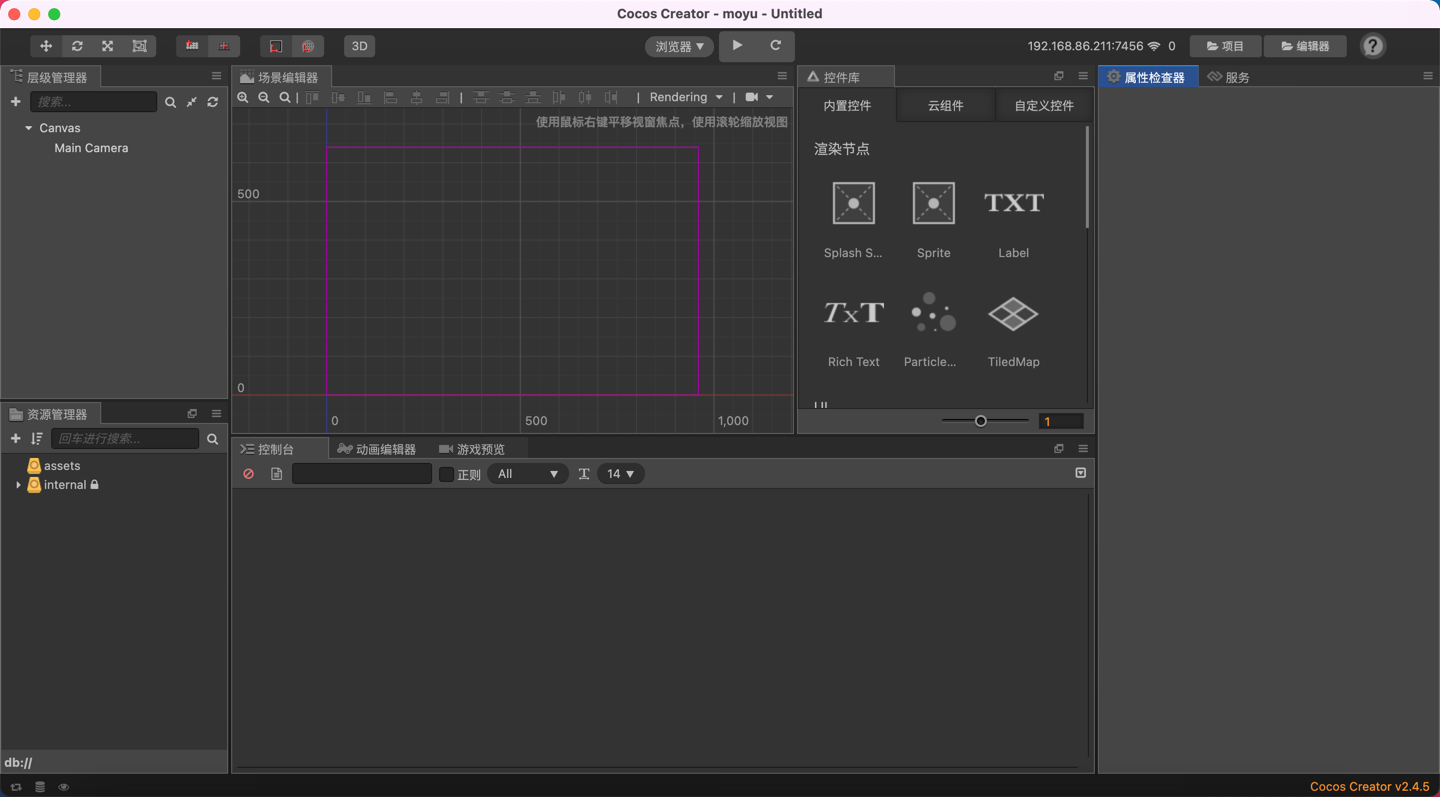## 3. 创建游戏画布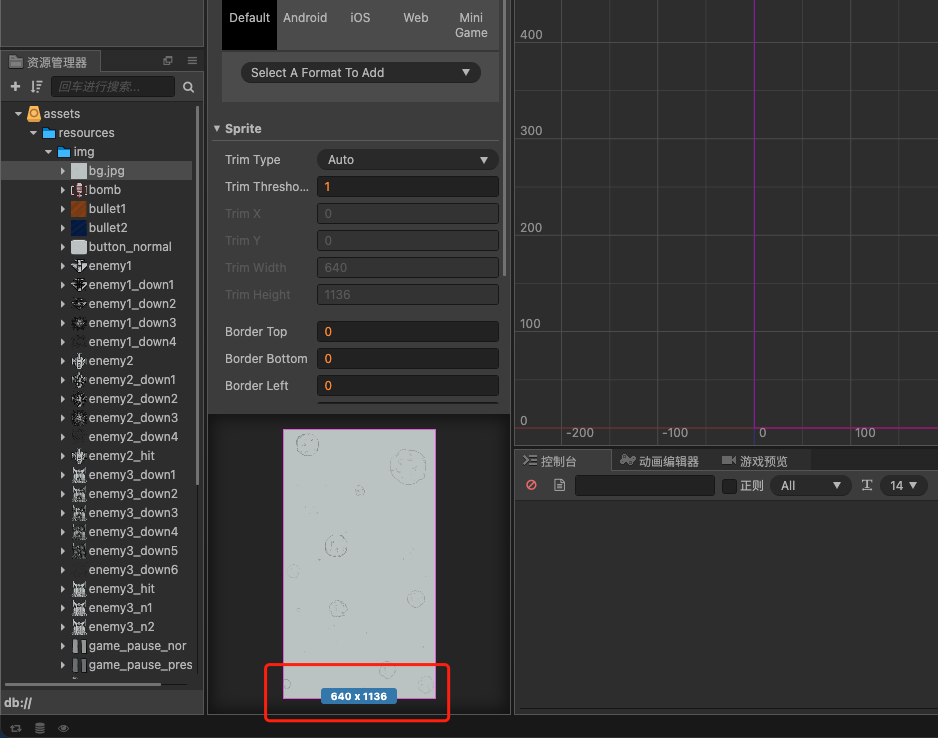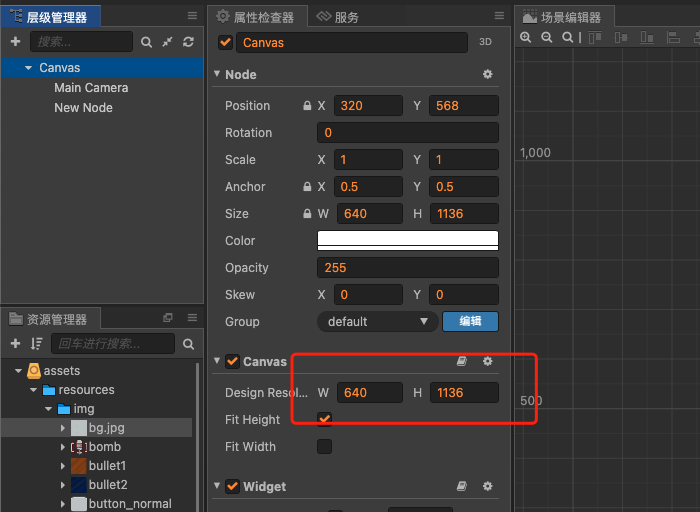## 4. 设置游戏背景

1. 打开图片目录，把所有的图片拖拽到 TexurePacker 中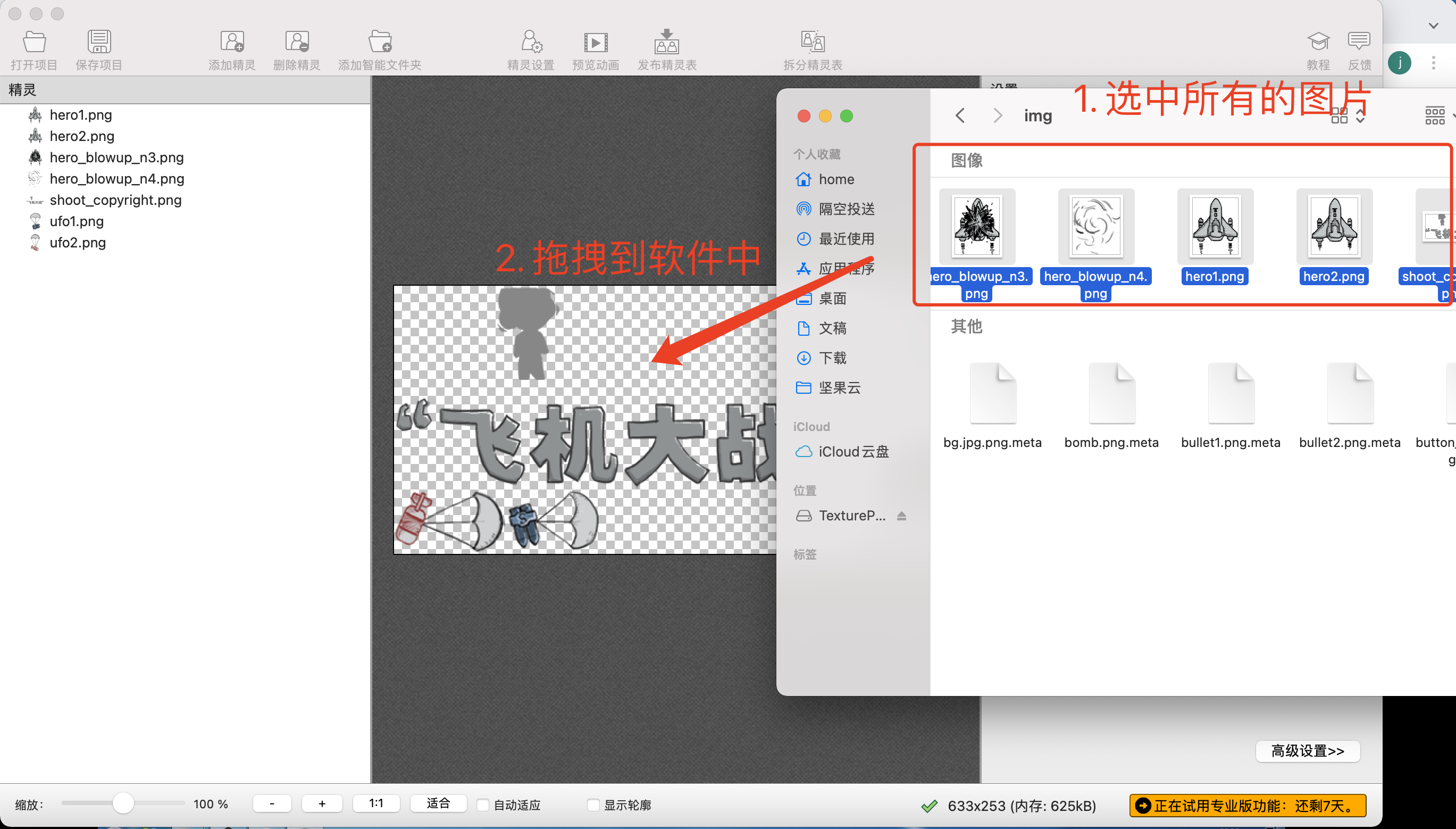1. 保存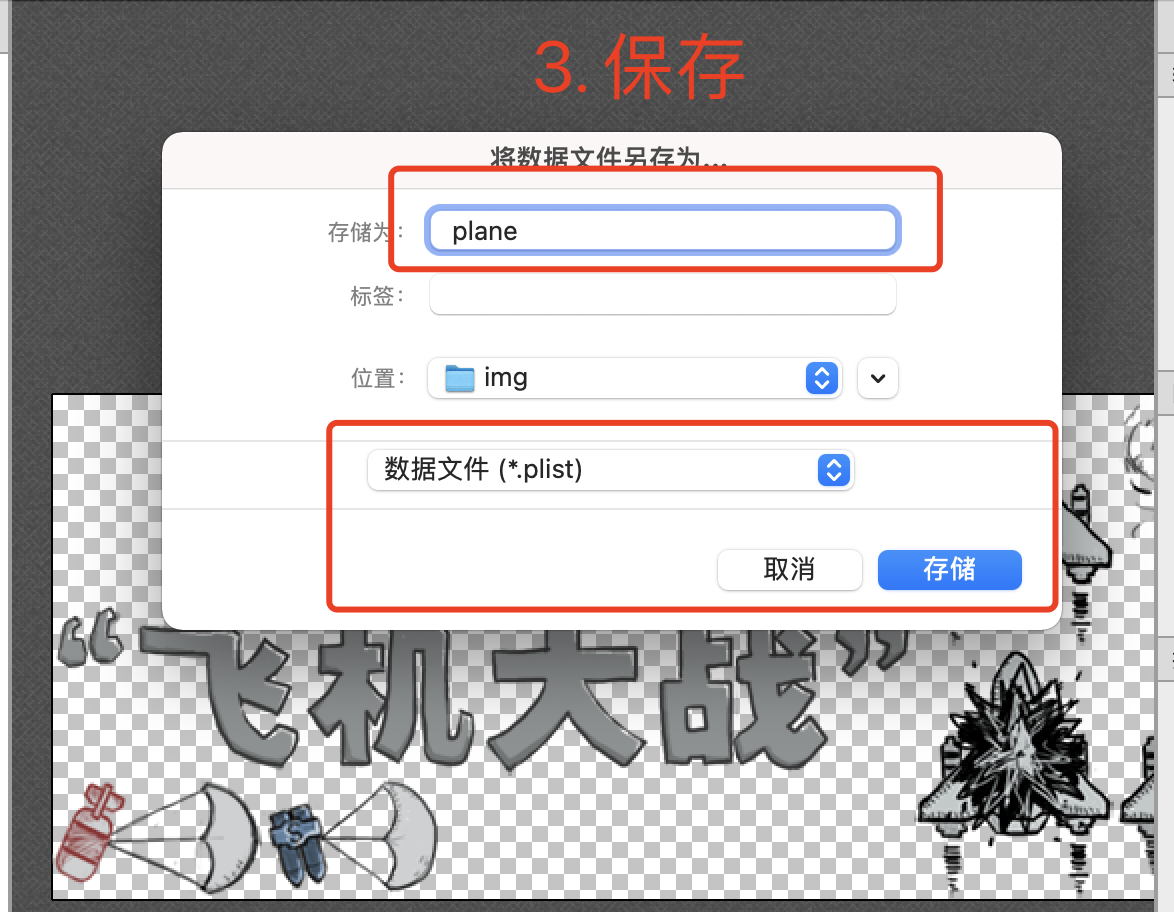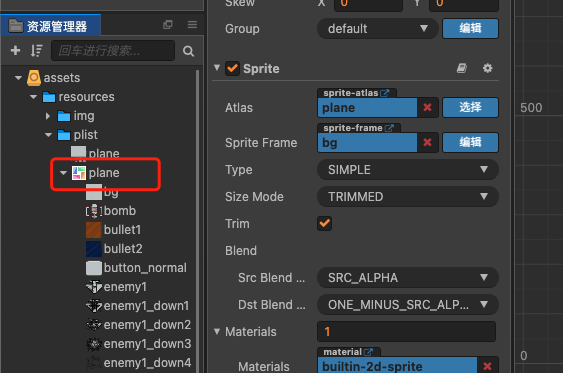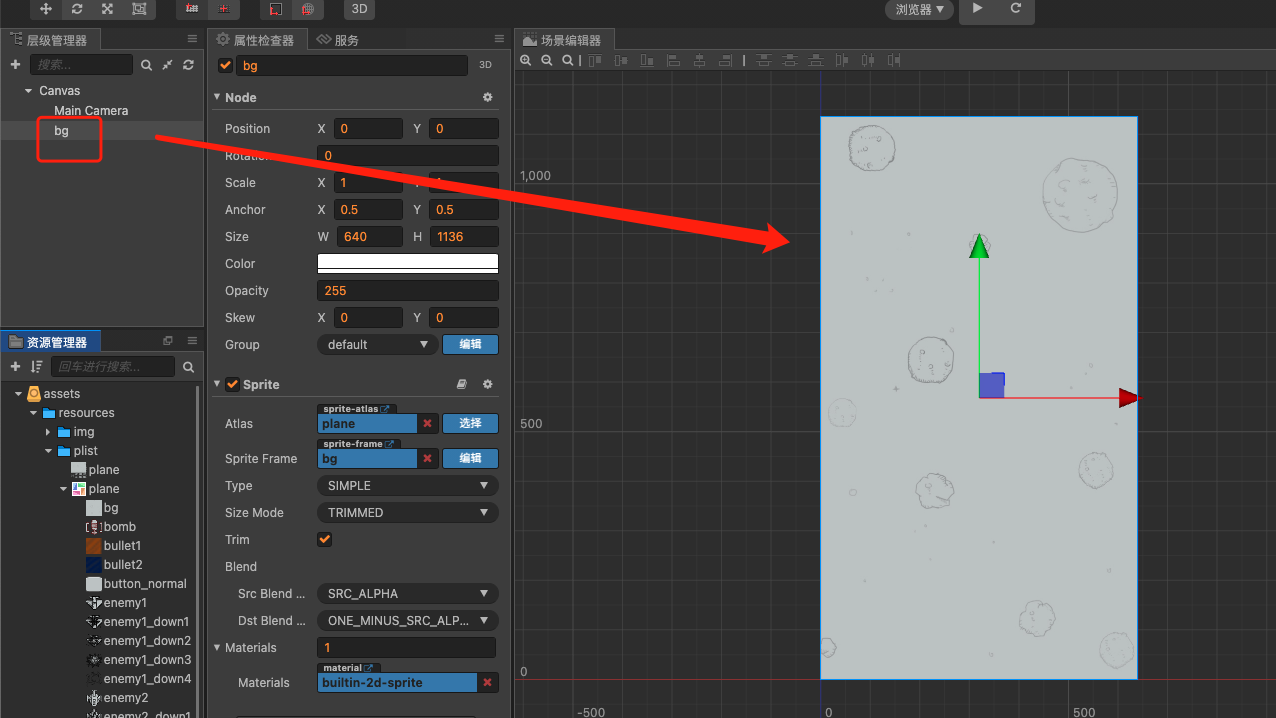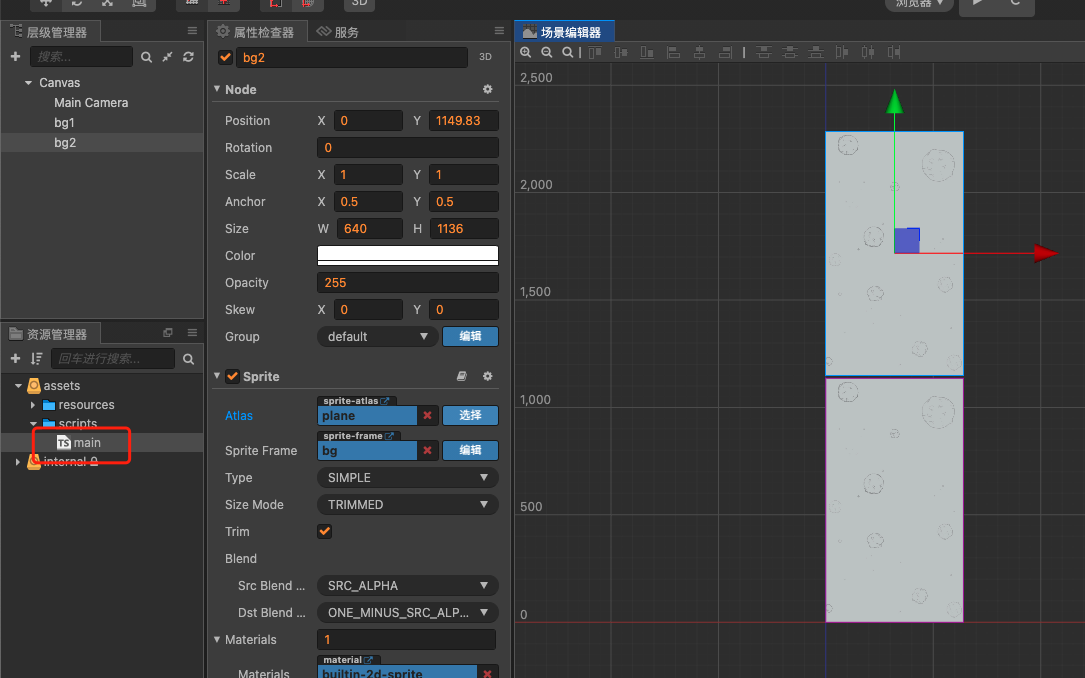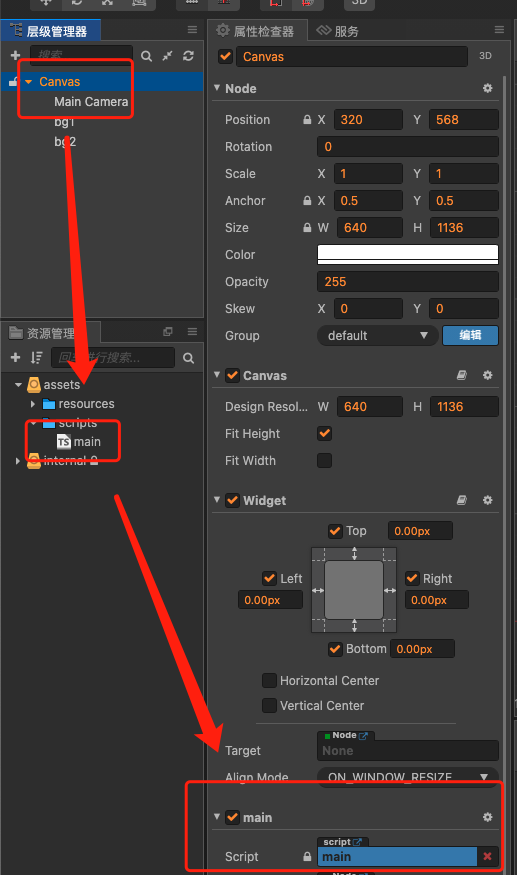``````@property(cc.Node)
bg1: cc.Node = null;

@property(cc.Node)
bg2: cc.Node = null;
``````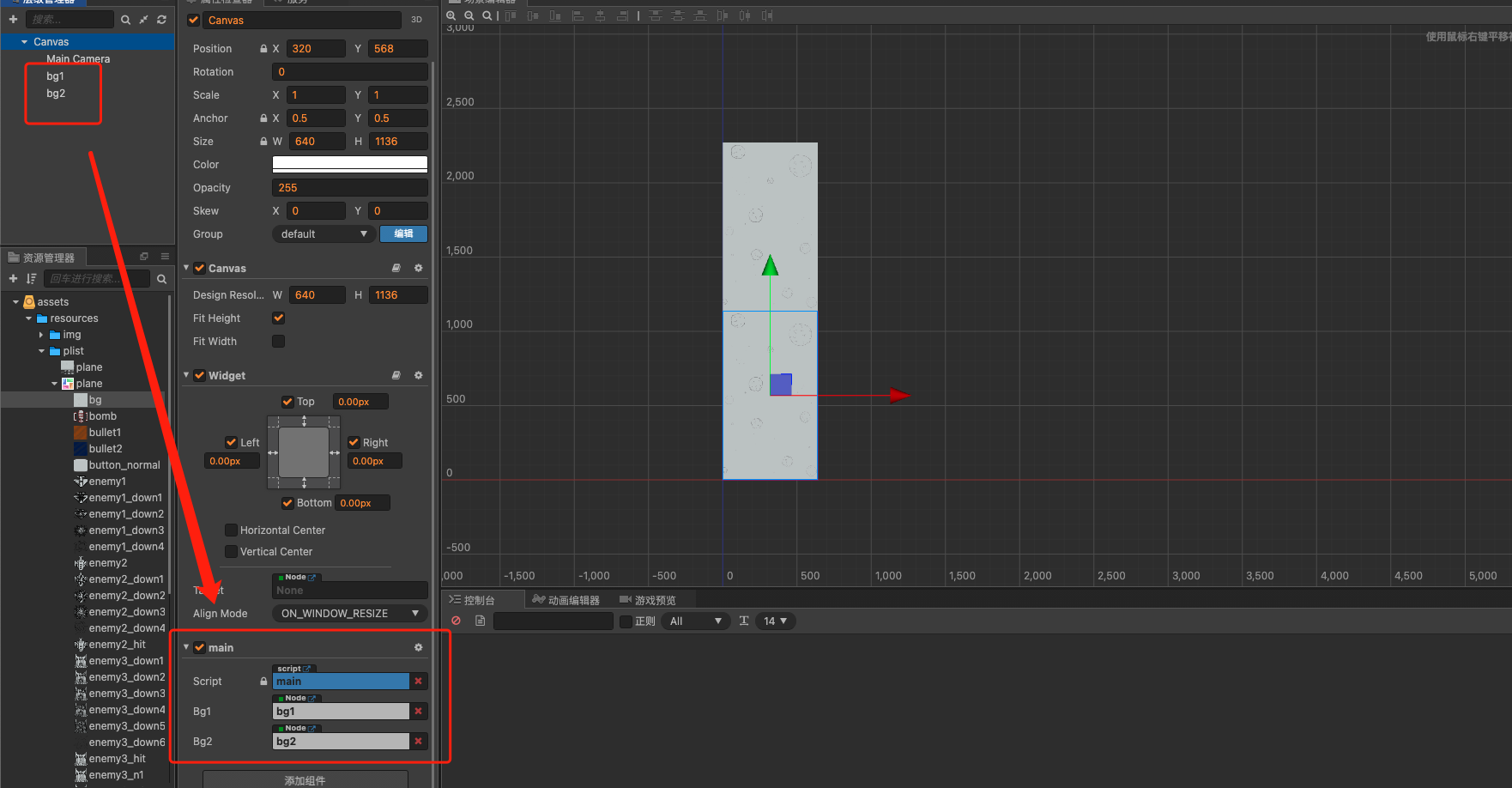``````// 在游戏加载的时候定义背景图片的位置
this.bg1.y = 0
this.bg2.y = this.bg1.y + this.bg2.height
}

update (dt) {
// 背景图片的移动速度
this.bg1.y -= 10;
this.bg2.y -= 10;
// 背景图片轮播逻辑（没明白可以看视频哦）
if(this.bg1.y <= -this.bg1.height){
// 当一张背景移动到屏幕外面后，立马补到另一张背景图片的后面
this.bg1.y = this.bg2.y + this.bg1.height
}
if(this.bg2.y <= -this.bg2.height){
this.bg2.y = this.bg1.y + this.bg2.height
}
}
``````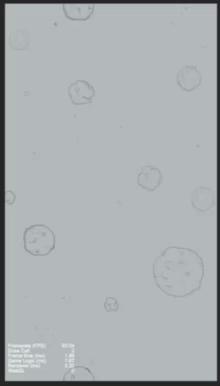## 5. 添加开始游戏标语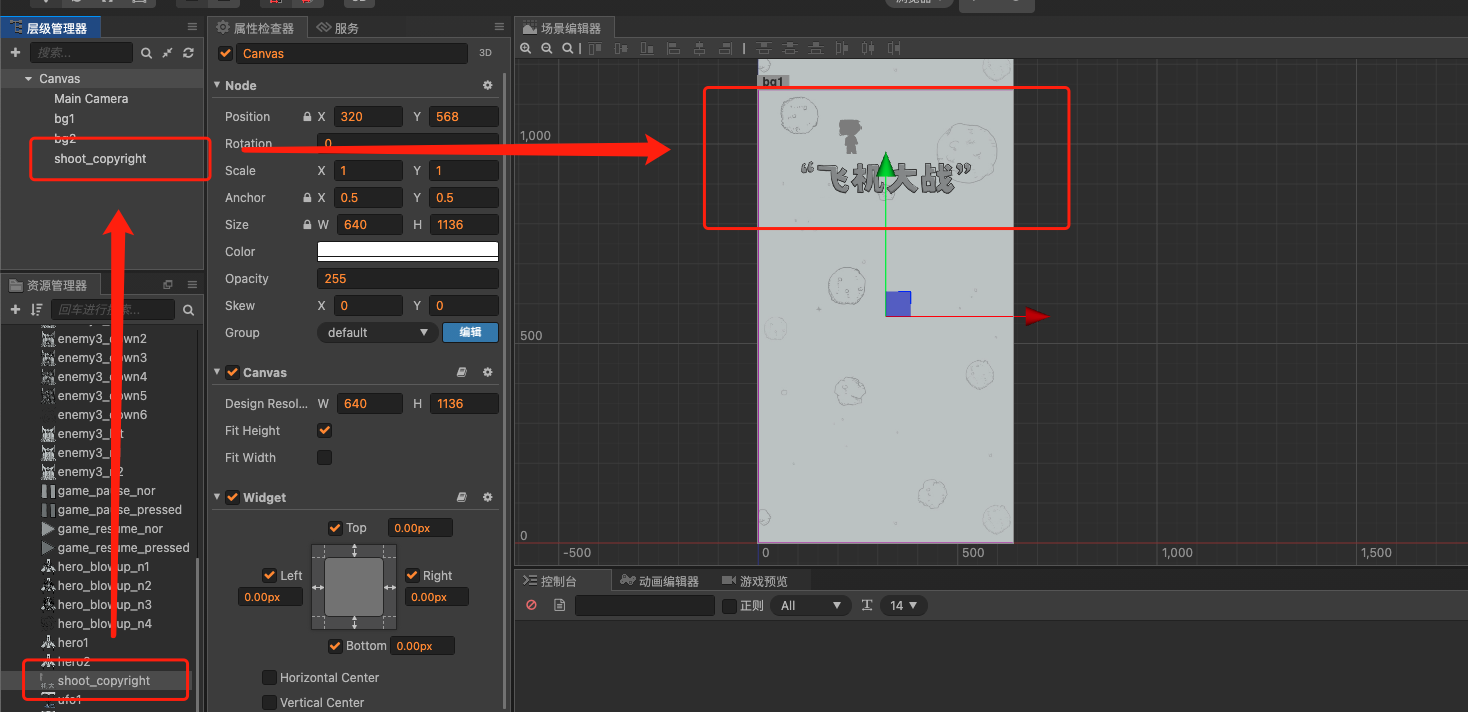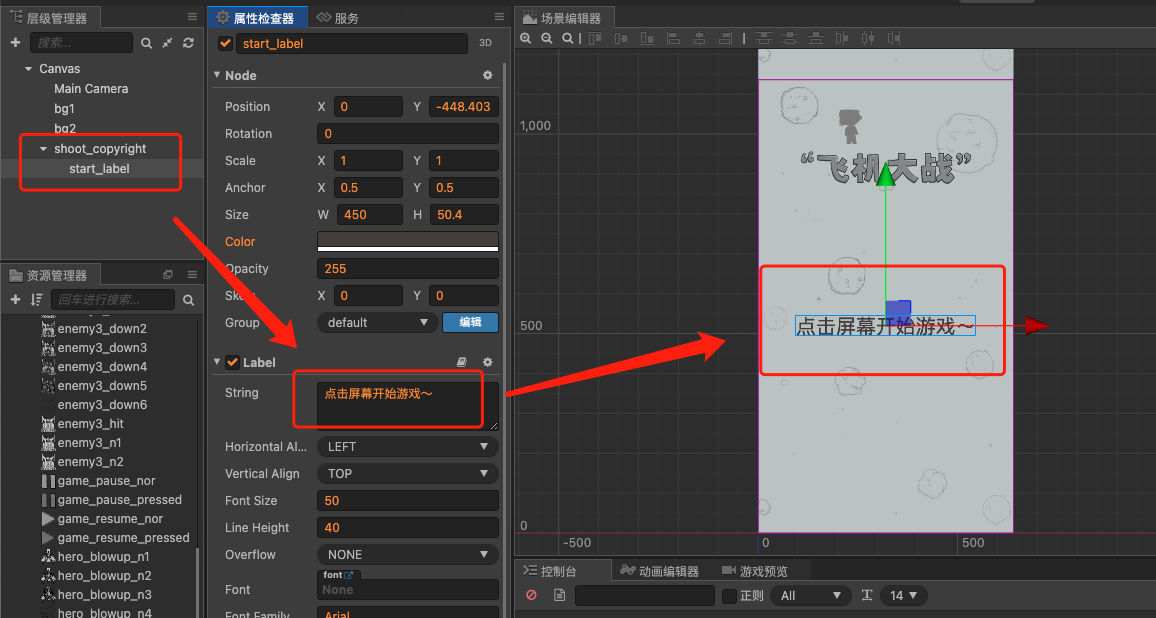1. 首先我们创建一个动画资源
2. 将动画资源绑定到 `点击屏幕开始游戏` 所在的节点上
3. 然后设置关键帧，给每个关键帧设定恰当的角度，比如说第一帧的角度为 0，第二帧的角度为 15，第三帧的角度为 0，第四帧为 -15，第五帧为 0。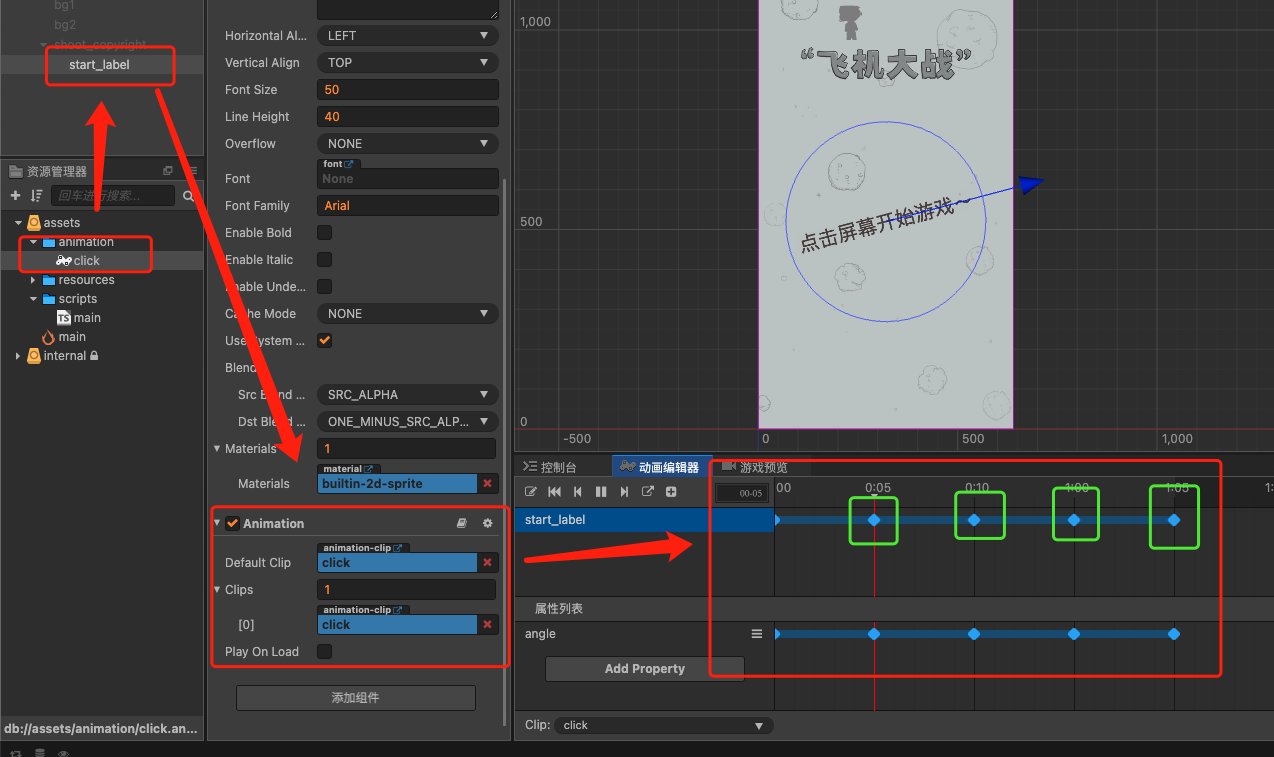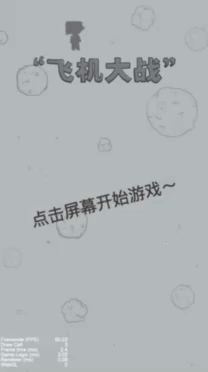## 6. 编写游戏准备、游戏中、游戏暂停三个状态

``````isBgMove = false
``````

``````update (dt) {
if(this.isBgMove){
this.moveBg()
}
}

moveBg(){
// 让背景图片动起来
this.bg1.y -= 10;
this.bg2.y -= 10;
if(this.bg1.y <= -this.bg1.height){
this.bg1.y = this.bg2.y + this.bg1.height
}
if(this.bg2.y <= -this.bg2.height){
this.bg2.y = this.bg1.y + this.bg2.height
}
}
``````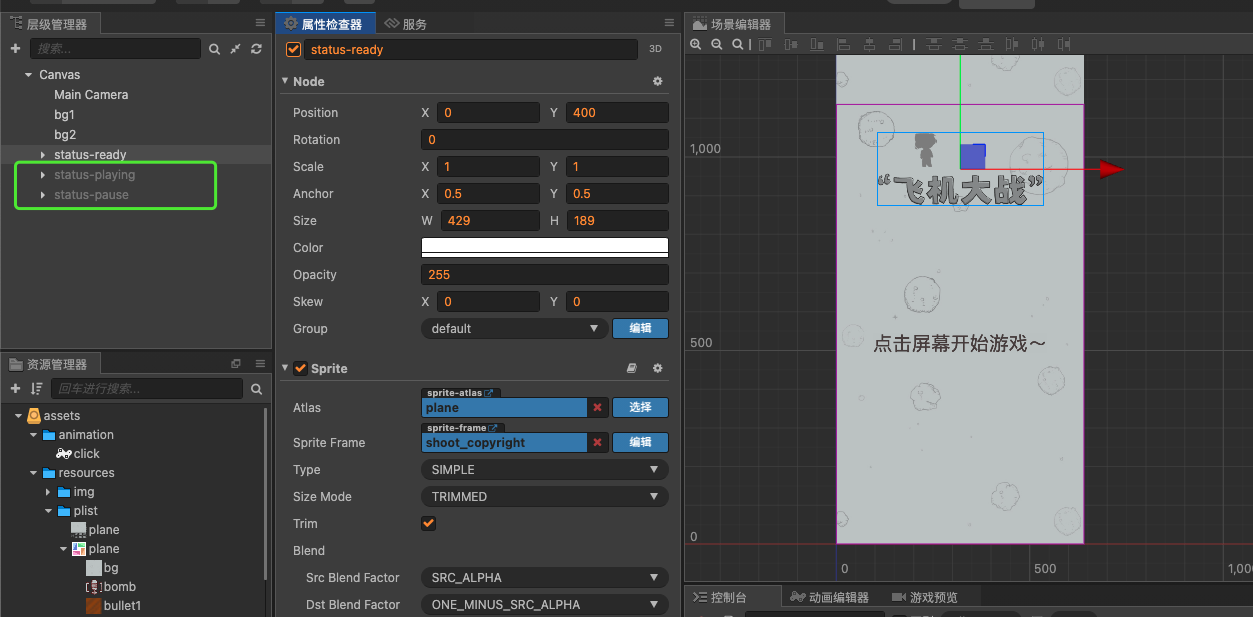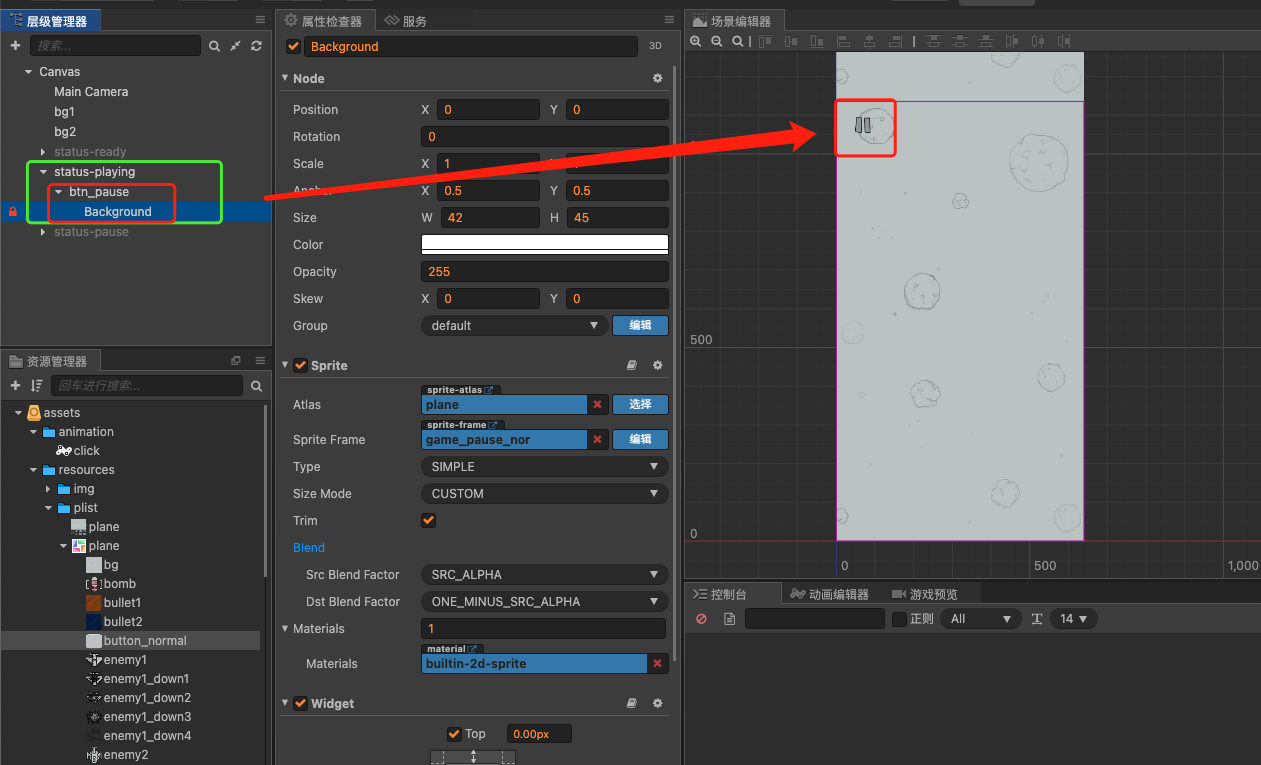``````clickButton(sender, str){
if(str == "pause"){
console.log("点击了暂停按钮")
}
}
``````

1. 首先添加一个暂停的背景图，设置透明度遮盖原有的游戏背景
2. 添加三个按钮，设置好按钮的样式以及显示内容
3. 为暂停背景设置一个 BlockInputEvents，屏蔽调下层节点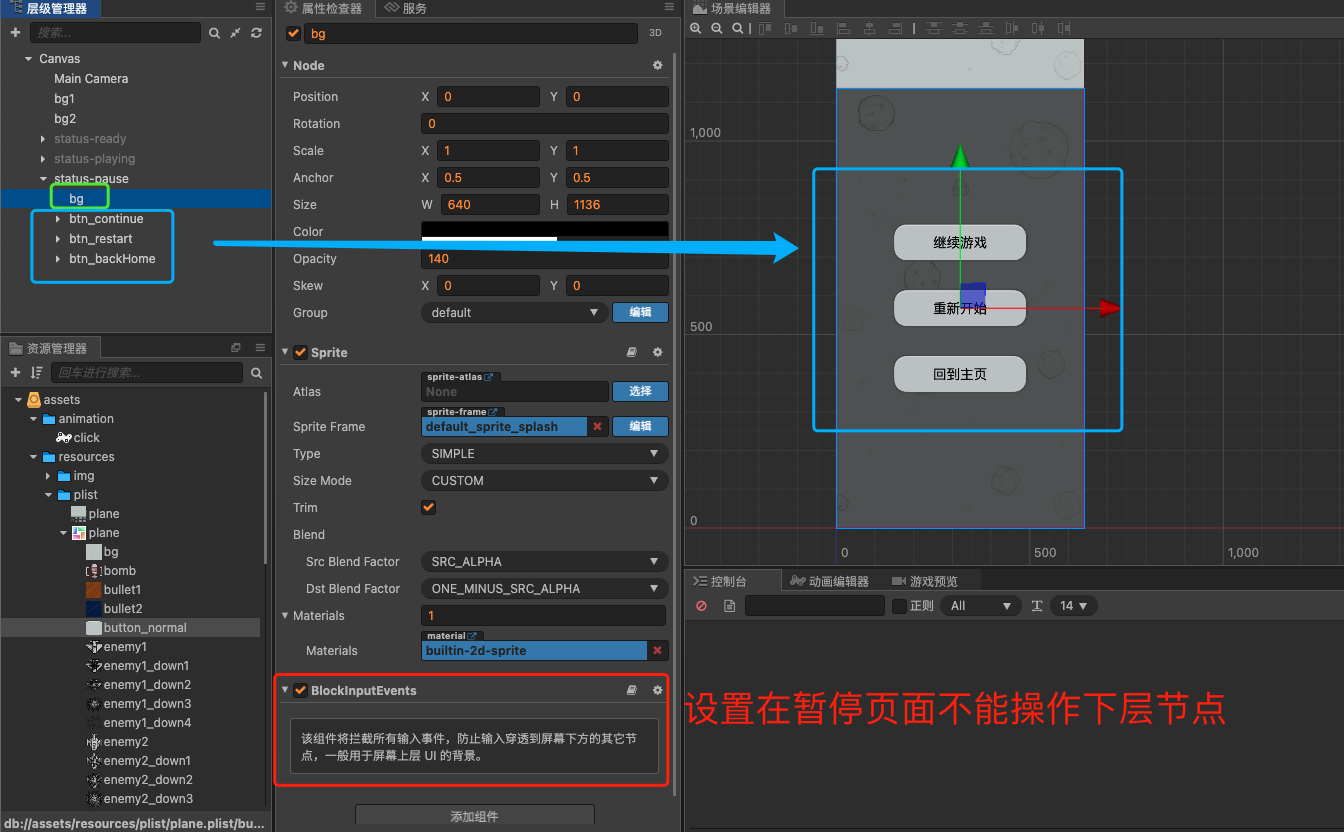1. 玩家进入游戏后显示一个静态的背景，当点击屏幕后隐藏调游戏准备界面，进入游戏开始界面
2. 玩家可以在游戏界面点击暂停按钮，点击暂停按钮后由游戏开始界面进入游戏暂停界面
3. 在游戏暂停界面有三个按钮，点击继续游戏会回到游戏开始界面，点击重新开始也会回到游戏开始界面，点击回到主页会回到游戏准备界面

``````clickButton(sender, str){
if(str == "pause"){
// 点击暂停后显示暂停页面
this.pause.active = true
}else if(str == "continue"){
// 点击继续游戏后隐藏暂停页面
this.pause.active = false
}else if(str == "restart"){
// 点击重新开始后隐藏暂停页面
this.pause.active = false
}else if(str == "backHome"){
// 点击回到主页隐藏暂停界面，停止游戏，停止背景移动
this.pause.active = false
this.playing.active = false
this.isBgMove = false
}
}
``````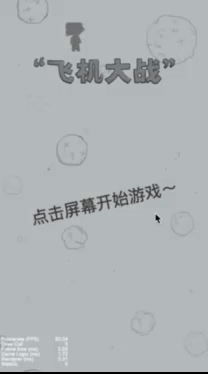## 7. 编写游戏主角我方飞机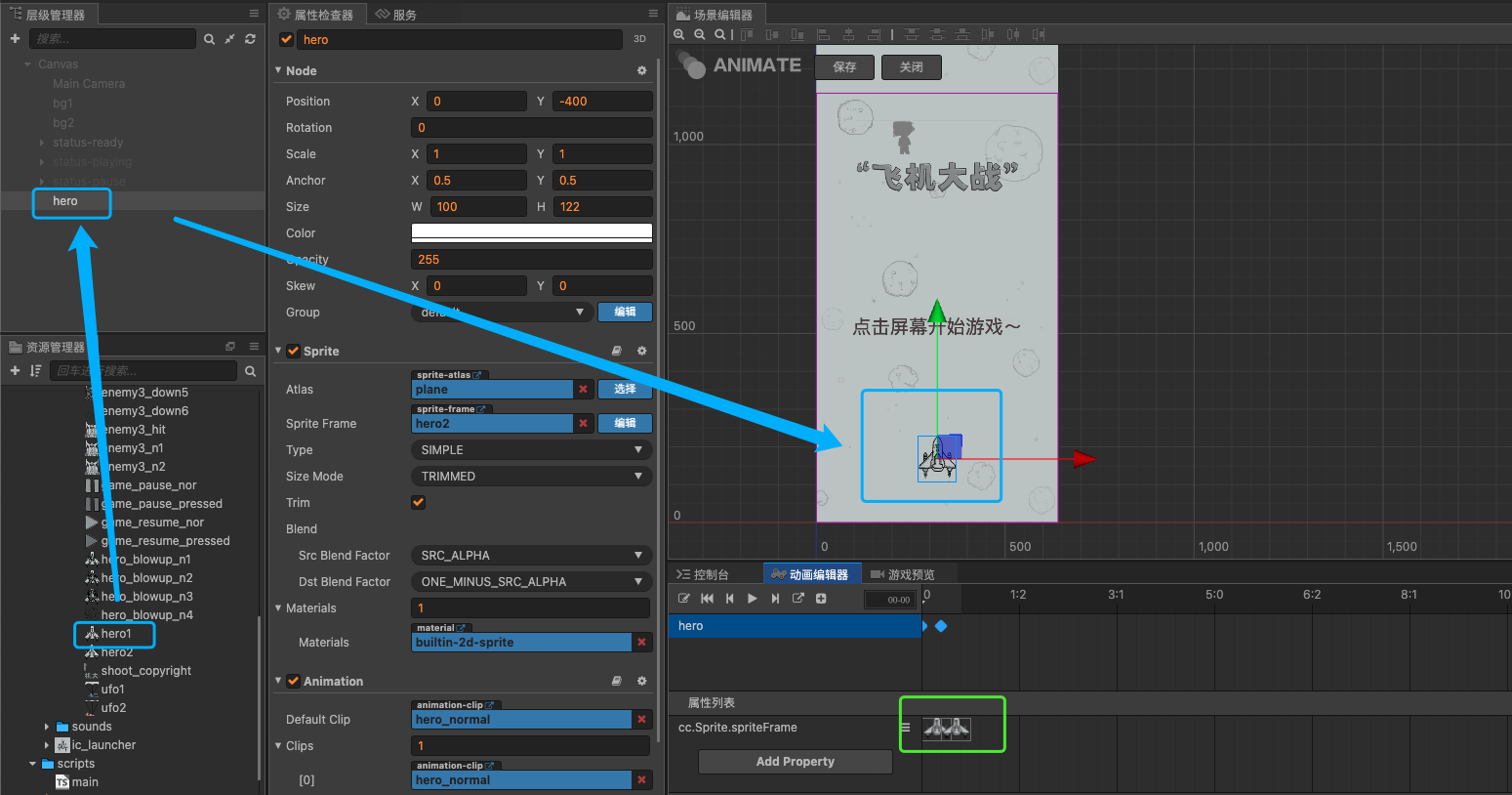``````setTouch() {
// ....
this.node.on("touchmove", (event) => {
// 获取飞机的位置
let hero_pos = this.hero.getPosition()
// 获取手指（鼠标）距离上一次事件移动相对于左下角的距离对象
let move_pos = event.getDelta()
// 飞机的位置加上移动的相对位置得到飞机的最新位置
this.hero.setPosition(cc.v2(hero_pos.x + move_pos.x, hero_pos.y + move_pos.y))
}, this);
//...
}
``````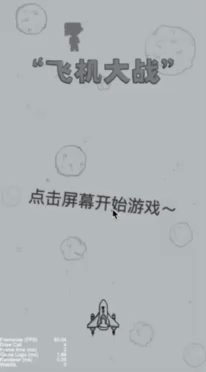## 8. 让飞机可以发射子弹

``````update(dt) {
this.node.y += 10
}
``````

``````// 子弹
@property(cc.Prefab)
pre_bullet: cc.Prefab
``````

``````setTouch() {
this.node.on("touchend", (event) => {
//......
// 生成一颗子弹
let bullet = cc.instantiate(this.pre_bullet)
// 把子弹挂在到节点树上
bullet.parent = this.node
// 获取飞机主角的位置
let pos = this.hero.getPosition()
// 设置子弹的初始位置为飞机头
bullet.setPosition(cc.v2(pos.x, pos.y + this.hero.height / 2))
}, this);
}
``````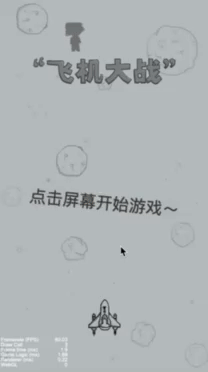## 9. 对象池与单例

``````createBullet() {
// 创建子弹的方法
let bullet = null
// 生成子弹的时候先到对象池中取
if (this.bulletPool.size() > 0) {
// 如果对象池中有子弹对象则直接使用
bullet = this.bulletPool.get()
} else {
// 如果对象池没有子弹了，就创建一颗新的子弹
bullet = cc.instantiate(this.pre_bullet)
}
// 获取子弹后挂在跟节点下
bullet.parent = this.node
// 获取飞机的位置
let pos = this.hero.getPosition()
// 设置子弹的初始位置
bullet.setPosition(cc.v2(pos.x, pos.y + this.hero.height / 2))
}
``````

1. 重新开始游戏
2. 回到主页
3. 子弹超出画布范围
``````// 回收单颗子弹
bulletKilled(bullet) {
// 回收子弹的方法
bullet.setPosition(cc.v2(0, 0))
this.bulletPool.put(bullet)
}

// 回收全部子弹
removeBullets() {
let children = this.node.children
for (let i = children.length - 1; i >= 0; i--) {
let bullet = children[i].getComponent("bullet")
if (bullet) {
this.bulletKilled(children[i])
}
}
}
``````

``````@ccclass
export default class Singleton extends cc.Component {

// 单例
public static instance: Singleton = null

// 初始化单例
if (Singleton.instance == null) {
Singleton.instance = this
} else {
this.destroy()
return
}
``````

``````const {ccclass, property} = cc._decorator;
// 导入主逻辑类
import Singleton from "./main";

@ccclass
export default class NewClass extends cc.Component {

update(dt) {
this.node.y += 15
if(this.node.y > 590){
// 使用主逻辑单例对象
Singleton.instance.bulletKilled(this.node)
}
}
}
``````

## 10. 添加敌机

1. 在节点树中创建一个敌机节点
2. 创建敌机的脚本，并关联给敌机节点
3. 把敌机节点制作成 PerFab
4. 在主逻辑中编写敌机对象池、敌机创建、敌机销毁的方法
``````createEnemy1() {
// 创建敌机1的方法
let enemy1 = null
if (this.enemy1Pool.size() > 0) {
enemy1 = this.enemy1Pool.get()
} else {
enemy1 = cc.instantiate(this.pre_enemy_1)
}
enemy1.parent = this.node
enemy1.setPosition(cc.v2(0, 590))
}

enemy1Killed(enemy1){
this.enemy1Pool.put(enemy1)
}

removeEnemy1s() {
let children = this.node.children
for (let i = children.length - 1; i >= 0; i--) {
let enemy1 = children[i].getComponent("enemy1")
if (enemy1) {
this.bulletKilled(children[i])
}
}
}
``````

[外链图片转存失败,源站可能有防盗链机制,建议将图片保存下来直接上传(img-jujgCh49-1647963420444)(https://s21.aconvert.com/convert/p3r68-cdx67/6d7lo-6fcx8.gif)]

## 11. 子弹与敌机碰撞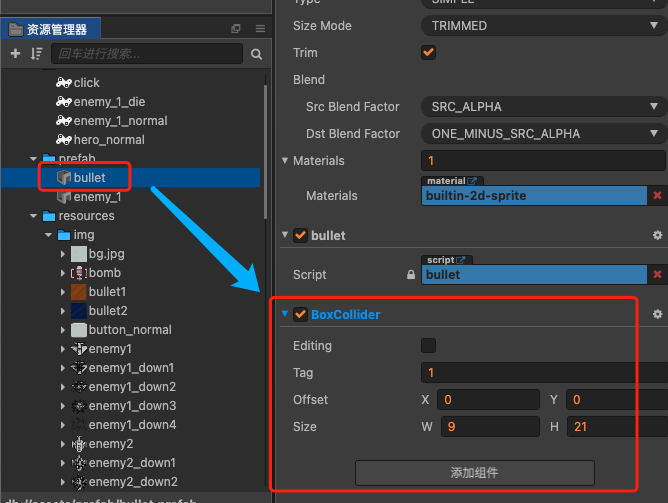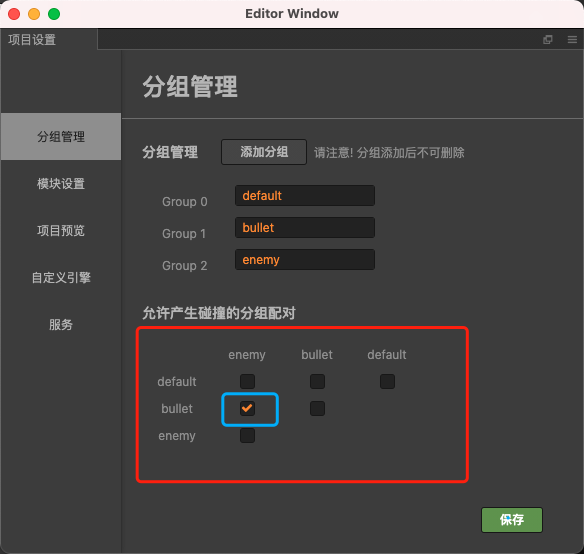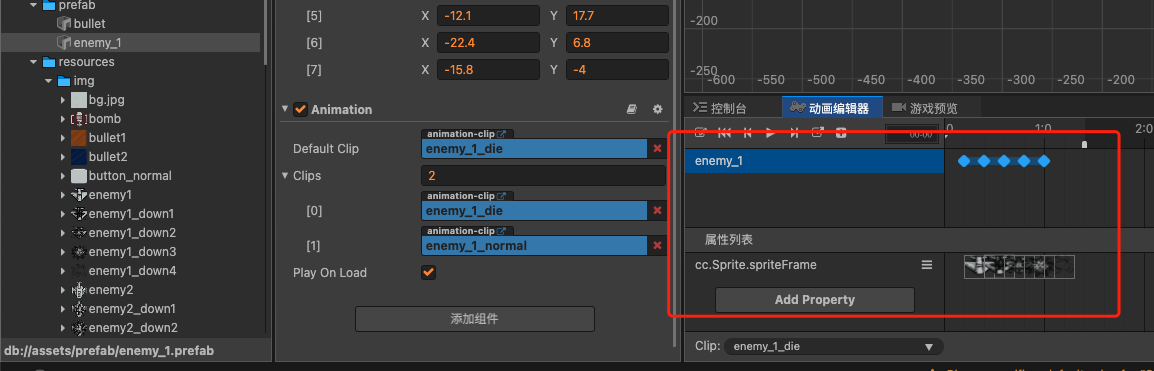``````// 开启碰撞检测系统，未开启时无法检测
cc.director.getCollisionManager().enabled = true;
``````

``````onCollisionEnter(other, self) {
if (self.tag == 1) {
// 普通子弹命中了普通敌机
Singleton.instance.bulletKilled(this.node)
}
if (other.tag == 2){
// 击中的是普通敌机
let enemy = other.getComponent("enemy_1")
if(enemy && !enemy.isDie){
enemy.hit()
}
}
}
``````

``````hit(){
// 击中后状态设置为死亡
this.isDie = true
// 播放帧动画
let anim = this.getComponent(cc.Animation)
anim.play('enemy_1_die')
}

over(){
// 帧动画播放完后把敌机放回对象池中，等待下一次出现
Singleton.instance.enemy1Killed(this.node)
}
``````

``````//...
enemy1.parent = this.node
let randomX = 295 - 590 * Math.random()
enemy1.setPosition(cc.v2(randomX, 590))
//...
``````